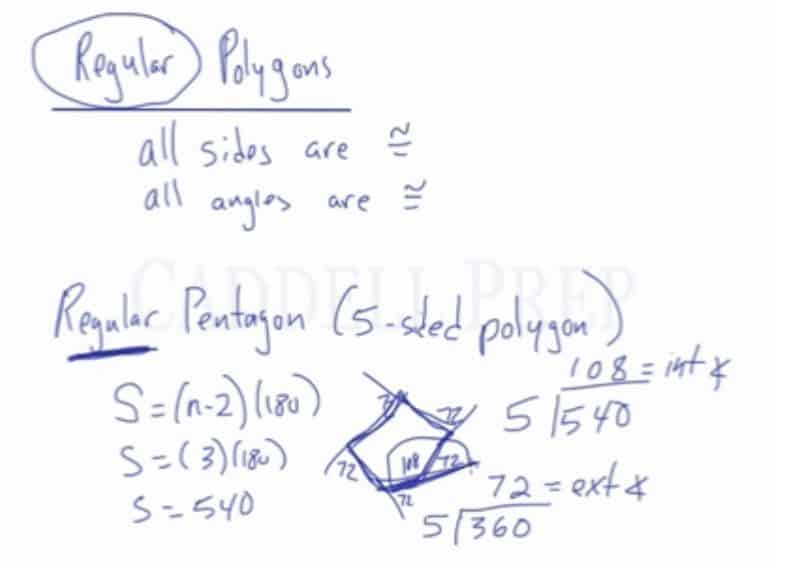In this video, we are going to look at the properties of interior and exterior angles of convex polygons, including how to calculate the sum of interior angles and the sum of exterior angles.

The number of exterior angles always equals the number of sides. The sum of the exterior angles is always$360\textdegree$, and that is true for all polygons.

The sum of interior angles differs by the number of sides polygons have.

• Triangles – There are 3 sides and a sum of 180 degrees
• Quadrilaterals – There are 4 sides and a sum of 360 degrees
• Pentagons – There are 5 sides and a sum of 540 degrees
• Hexagons – There are 6 sides and a sum of 720 degrees

Notice that every time we add a side, the sum of the angles increases by 180. There is a formula for this:$S=(n-2)(180)$
where S is the sum of the angles and n is the total number of sides.

Regular polygons: All sides and all angles are congruent.

After knowing the total sum of the interior angles, we can divide by the number of angles to determine the degree measure of each individual angle. We can also do this for the degree measures of the exterior angles, by dividing the 360 by the total number of angles.Video-Lesson Transcript

Let’s take a look at angle relationships in polygons.

First of all, a polygon is any shape with three or more sides.

Let’s look at the exterior angles.

Exterior angles are created by extending all the sides in one direction.

Here, we have six exterior angles and six sides.

The number of exterior angles always equal sides.

Let’s label the exterior angles as$a^{\circ}$,$b^{\circ}$,$c^{\circ}$,$d^{\circ}$,$e^{\circ}$, and$f^{\circ}$.

The sum of all these exterior angles is always equal to$360$.$a + b + c + d + e + f = 360$

That is true for all polygons.

For triangles, squares, trapezoids, hexagons, pentagons, and octagons. It doesn’t matter. Exterior angles always add up to$360$.

On the other hand, the sum of interior angles will be different depending on how many sides there are.

For a triangle, which we know has three sides, the sum of interior angles is$180$.

Quadrilaterals such as a square or parallelogram which has four sides, angles add up to$360$.

In a five-sided figure, a pentagon, the sum of the angles is$540$.

A hexagon is a six-sided figure, the sum of its interior angles is$720$.

You should notice that every time we add a side, the sum of the angles go up by$180$.

So, if you want to know the sum of the interior angles of a certain polygon, just make a quick chart starting with a triangle at$180$ and keep on adding$180$ as you increase the sides.

But there’s a formula for this.$S = (n - 2) (180)$

where$S$ is the sum of interior angles and$n$ is the number of sides

For example, we have a five-sided figure:$S = (n - 2) (180)$$S = (5 - 2) (180)$$S = (3) (180)$$S = 540$

just like what we had in our chart

This is the formula for finding the sum of the interior angles. And the other one is the chart version.

And the sum of all the exterior angles of any polygon always adds up to$360$.

Now, let’s go over is a regular polygon.

Regular means all sides are congruent. This means that all the sides are of the same length. All angles are also congruent.

If we have a regular pentagon, which is a five-sided polygon, we know what the sum of the interior angles going to be.

We can simply find the sum using the formula$S = (n - 2) (180)$$S = (3) (180)$$S = 540$

And because it is a regular pentagon, all the interior angles are the same.

We can find out how big each of its angles will be.

Simply divide$540$ evenly among the five angles.

The answer is$108$.

So, each interior angle is$108^{\circ}$.

We can also find the exterior angle.

One straight line has to be$360$, so if the interior angle is$108$, then the exterior angle is$72^{\circ}$.

Another way to do it is to know that all the exterior angles of any polygon have to add up to$360$.

We can do$360 \div 5 = 72$

So,$72$ is the exterior angle. Each exterior angle is$72^{\circ}$.

Since we know that an exterior angle is$72$ and one straight line is$180$, then the interior angle is$108$.